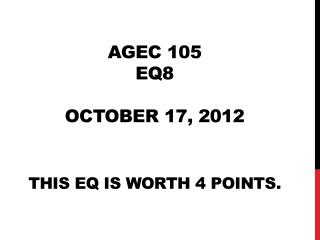# AGEC 105 EQ8 October 17, 2012 This EQ is worth 4 points. - PowerPoint PPT PresentationDownload PresentationAGEC 105 EQ8 October 17, 2012 This EQ is worth 4 points.

AGEC 105 EQ8 October 17, 2012 This EQ is worth 4 points.
Download Presentation## AGEC 105 EQ8 October 17, 2012 This EQ is worth 4 points.

- - - - - - - - - - - - - - - - - - - - - - - - - - - E N D - - - - - - - - - - - - - - - - - - - - - - - - - - -
##### Presentation Transcript

1. AGEC 105EQ8October 17, 2012This EQ is worth 4 points.

2. Question 1 (1pt) Given the market supply schedule below: Calculate the own-price elasticity of supply between points C and D. Show all work.

3. Question 2 (2pt) Suppose that P = \$25/unit and the profit maximizing output is 350 units. Also suppose that the corresponding ATC = \$16/unit. Calculate the maximum profit that this firm can earn. Show all work.

4. Question 3 (1pt) Consider the following diagram: On the basis of this diagram, producer surplus is equal to \$___________ million. P S \$4 \$2 Q (million) 30